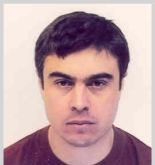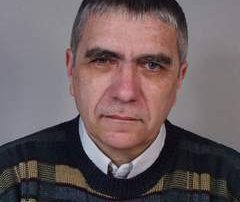•Theoretical and mathematical physics, Physics of space-time and gravity, Astrophysics and cosmology, Differential geometry and topology, Non-linear private differential equations, Numerical methods and computational physics

2018-09-17T21:40:13+03:00Sunday, 15 July 2018|
•## Chobanov, Georgi

Nonlinear Partial Differential Equations, Topological methods in the theory of nonlinear equations, Analytic mechanics, Operational calculus.

2018-09-17T21:24:56+03:00Friday, 27 April 2018|
•## Topalov, Peter

Hamiltonian PDEs and ODEs, dynamical systems, Riemannian and symplectic geometry, billiards.

2018-09-17T21:24:48+03:00Friday, 27 April 2018|
•## Tintschev, Vassil

2018-09-17T21:25:04+03:00Thursday, 26 April 2018|
•## Tarulli, Mirko

Equations of Mathematical physics, Nonlinear PDEs, Scattering theory.

2018-09-17T21:31:12+03:00Thursday, 26 April 2018|
•## Stefanov, Alexander

Nonlinear dynamical systems related to infinite dimensional Lie algebras.

2020-08-11T11:45:58+03:00Thursday, 26 April 2018|
•## Slavova, Angela

I am working in the theory of differential equations, more precisely in partial differential equations (PDE), CNN modeling of complex nonlinear partial differential equations arising from applications in mathematical physics, in biology, in ecology, in mechanics etc.

2020-08-11T11:45:34+03:00Saturday, 14 April 2018|
•## Rangelov, Tsviatko

Partial differential equations and applications: Solvability and hypoellipticity for pseudodifferential operators with multiply characteristics; Scattering theory for hyperbolic equations and systems for moving obstacles; Nonlinear degenerate parabolic equations; BIEM for systems of elasticity in the domains with cracks.

2018-09-17T21:25:09+03:00Saturday, 14 April 2018|
•## Popov, Georgi

Spectral theory, scattering theory, dynamical systems.

2020-05-18T13:52:18+03:00Friday, 13 April 2018|
•## Popivanov, Peter

The main research interests and publications of Peter Popivanov are in partial differential equations - linear and nonlinear micro-local analysis nonelliptic border problems and global solvability, hipoellipticity, propagation of singularities of weakly nonlinear hyperbolic equations and systems and applications in mechanics and geometry.

2018-09-17T21:25:24+03:00Friday, 13 April 2018|